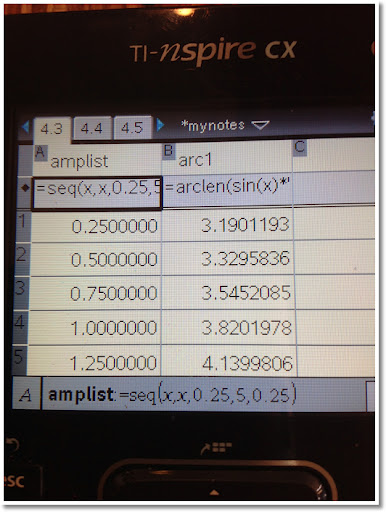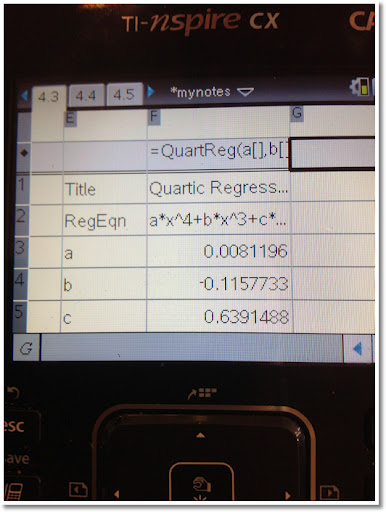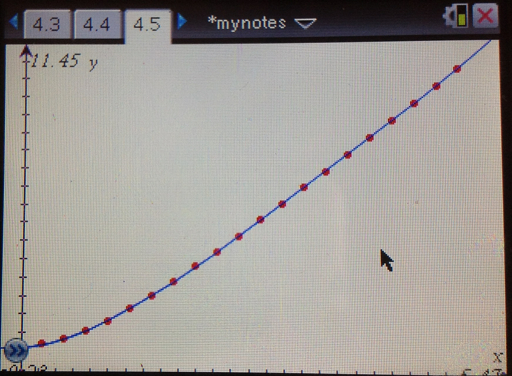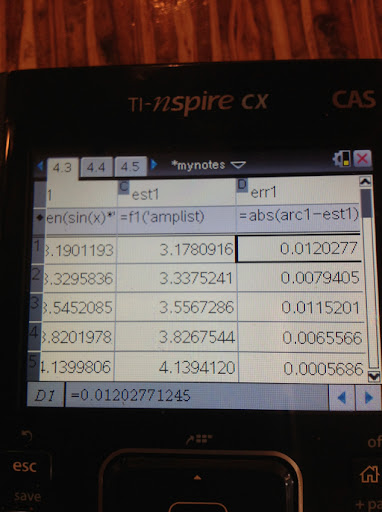## Monday, May 21, 2012

### Arc length of sin(x) - Curve Approximation

Blog Entry #102

The Arc Length of a Sine Curve

The sine curve is one the most interesting curves in mathematics.

Let

y = a * sin x ,

Where a is the amplitude of the sine curve.

We can find the arc length of a curve between limits x1 and x2 by the integral:

x2
∫ √ (1 + (dy/dx)^2) dx
x1

For the sine curve:

y = a sin x

dy/dx = a cos x

(dy/dx)^2 = a^2 cos^x

And the arc length is:

x2
∫ √(1 + a^2 cos^x) dx
x1

There is no anti-derivative for √(1 + a^2 cos^x). Therefore, numerical methods must be used.

Finding an Approximate Curve

Using a TI nSpire CX CAS, I used the Spreadsheet, curve fitting, and graphing features to determine an approximate polynomial. The arc length is from the origin (0,0) to (π, 0).

Note: x1 = 0, x2 = π

Here is a shot summary of what I did:1. Created a spreadsheet with the following columns:

Column A: A sequence of numbers from 0.25 to 5 in increments of 0.25. The resulting list is named amplist.

Column B: Use the nSpire's arcLen function to get the arc length of the sine curve using amplist as the values for a. This list is named arc1.2. Pressing the menu key allowed me to access the Statistics menu. Using the Stat Calculations option, I used different types of regression, including power and quartic regression. What I was looking for was which regression had the best coefficient of determination (R^2). In general, the closer R^2 is to 1, the better the fit.

I ended up choosing the quartic regression (4th degree polynomial) with R^2 ≈ 0.9999919.

The approximate polynomial is

y = .0081196317102889 x^4 - .11577326164517 x^3 + .63914882375794 x^2 + .2071162669684 x + 3.0881429428239

This polynomial was save to the function f1(x).3. I created a graphs page and made two plots:

* Scatter plot where x = amplist, y = arc1. (The dots in red)
* The function f1(x) (see step 2). (The curve in blue)

Setting zoom to fit the data, the curve looks like a good fit.

You can create a similar graph with the Data & Statstics module, but I thought I would be different this time.

How good of a fit is the polynomial?4. I went back to the spreadsheet and added two more columns.

Column C: est1 = f1(amplist). (estimate arc lengths)
Column D: err1 = abs(arc1 - est1)

By scrolling down Column D, the quartic polynomial was accurate in estimating the arc length of the sine curve from 0 to π to at least two decimal places.

Conclusion

We have been looking to find the arc length of the curve y = a sin x from x = 0 to x = π.

The exact value is:

π
∫ √ (1 + a^2 cos^2 x ) dx
0

However, a good estimate can be found (to 2-3 decimal places) with the polynomial:

y = .0081196317102889 x^4 - .11577326164517 x^3 + .63914882375794 x^2 + .2071162669684 x + 3.0881429428239

Thanks as always and talk to you soon!

Eddie

This blog is property of Edward Shore. © 2012

#### 1 comment:

1.Thanks. It's a nice post about arc length formula. I really like it :). It's really helpful. Good job.

### Casio fx-5800P and TI-84 Plus CE: Simplify Radicals and August TI-95 ProCalc Month

Casio fx-5800P and TI-84 Plus CE: Simplify Radicals and August TI-95 ProCalc Month Introduction The program SIMPRAD simplifies ra...